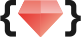# 五倍紅寶石 ・專業程式教育機構## 什麼是 DSL? DSL 的存在意義是什麼？

DSL 為 Domain Specific Language 的縮寫，中文譯為「特定領域語言」。而 DSL 的開發通常是為了迎合某些特殊的需求，例如針對系統、平台、程式工具、軟體問題、商業邏輯等等領域，當人們發現現有的程式語言並沒有辦法好好地嵌合使用需求，DSL 就有其存在的價值。目前為人所知的 DSL 包括 CSS 以及 SQL，而這種易於人類閱讀的 DSL 也能夠讓程式設計師乃至於一般使用者都能享受 DSL 帶來的好處，甚至不懂程式設計的使用者都能夠針對自己的需求調整參數。

## 測試範例

``````class CatFactory
def produce
"meow"
end
end

describe CatFactory
describe "#produce" do
it "produce a cat and meowing" do
expect(CatFactory.new.produce).to eq("meow")
end

it "does not produce a dog" do
expect(CatFactory.new.produce).not_to eq("woof")
end
end
end``````

## 實作 RSpec

``````class Describe
def initialize(context, &block)
@context = context
instance_eval(&block)
end

# 為了讓我們可以使用 describe 來做成巢狀結構
def describe(context, &block)
Describe.new(context, &block)
end
end``````

``````# helper method

def describe(context, &block)
Describe.new(context, &block)
end``````

``````class Example
def initialize(context, &block)
@context = context
instance_eval(&block)
end

def expect(result)
end

def to(expectation)
end

def not_to(expectation)
end

def eq(expectation)
end
end``````

#expect 這個函式單純只是保存測試的結果，因此是最為容易的一個。

``````  def expect(result)
@result = result

# 為了讓 #expect 之後可以繼續串 Example 裡頭的其他函數，所以我們這裡希望回傳 self。

self
end``````

#eq 這個函式很單純只是做出像 `==` 一樣的運算，不過我們必須將它傳進 #to，並且和真正的結果對比。這裡我們會用 Proc 來處理。

``````  def to(expectation)
@test_result = expectation.call(@result)
end

def eq(expectation)
Proc.new { |result| result.eql?(expectation) }
end``````

``````  def not_to(expectation)
@test_result = !(expectation.call(@result))
end``````

## 顯示測試的結果

``````  class Example

# other code below
# ...
end``````

``````  class Describe
attr_reader :context, :examples, :describes
def initialize(context, &block)
@context = context
@describes = []
@examples = []
instance_eval &block
end

def describe(context, &block)
describes << Describe.new(context, &block)
end

def it(context, &block)
@examples << Example.new(context, &block)
end
end``````

``````    def test
puts context
describes.each do |describe_node|
puts "  " + describe_node.context
describe_node.examples.each do |example_node|
color = example_node.test_result ? :green : :red
puts "    " + example_node.context.colorize(color)
end
end
end``````

``````rspec = describe CatFactory
describe "#produce" do
it "produce a cat and meowing" do
expect(CatFactory.new.produce).to eq("meow")
end

it "does not produce a dog" do
expect(CatFactory.new.produce).not_to eq("woof")
end
end
end

rspec.test
``````

``````CatFactory
#produce
produce cat and meowing
does not produce dog``````

## 總結

👩‍🏫 課務小幫手：

✨ 想掌握 Ruby on Rails 觀念和原理嗎？